Vibroengineering PROCEDIA

Published: 13 June 2022

# Dynamical stability analysis for a micro shell subjected to swirling annular flow including the coupling effect of small size and fluid rotation

Cheng Chen1
Xinlei Chen2
Wenbo Ning3
Junzhang Wu4
Yuehong Liu5
1, 2, 3, 4Jiangsu Key Laboratory of Advanced Manufacturing Technology, HuaiYin Institute of Technology, Huai’an, China
5Huaian Weili Pump Technology Co., Ltd, Huai’an, China
Corresponding Author:
Cheng Chen
Views 14

#### Abstract

This paper is concerned with stability analysis for an outer thin-walled shell conveying a swirling flowing fluid in the annular space between the inner shell and outer shell with micro-dimensions. It describes the development of theoretical model in the framework of the modified coupled stress theory along with the Donnell shell theory. The theoretical model uses the Hamilton’s principle to derive the governing motion equations of the shell. The fluid is assumed to be incompressible and irrotational and the hydrodynamic pressure is considered with the version of the potential flow theory. The zero-level contour method is utilized to display the complex dynamical behaviors of micro shell subjected to swirling annular flow. The effects of the material intrinsic parameter, the fluid rotation on stabilities of the fluid microscale shell system are discussed fully. The results elucidate that the coupling effect of the fluid rotation and the material intrinsic parameter on such system.

## 1. Introduction

As a fundamental structural element, macro-scale shells/tubes containing flowing fluid are encountered in many engineering applications. A remarkable number of scientists and engineers have made great efforts on different aspects of the topic. Especially, dynamical behaviors of coaxial cylindrical shells conveying axial flow have been considered [1, 2], and later the further work of shells conveying helical annular flow was performed .

Owing to low power, super mechanical and electrical properties, micro/nano structures such as shells/tubes have become more important in the past fifty years. The classical continuum mechanics cannot predict size-dependent responses of the microstructures. To explain the size dependent mechanical behaviors of such microstructures, some outstanding researchers have developed the nonlocal elasticity theory/couple stress theory /strain gradient theory, in which the modified couple stress theory  has been used widely because of its symmetric form and the involvement of only one material length scale parameter. Based on the Differential Quadrature Method (DQM), the dynamical responses of fluid-conveying microtubes were investigated by Wang  from the modified coupled stress theory. The threshold values of instability are higher because the modified couple stress theory considered the size effects. The nonlinear studies of fluid-conveying micro pipes was studied by the literature . It was found that the material intrinsic parameter causes micro pipes more stable. And complex vibrations could be observed when the micropipe boundary meets certain conditions. Based on the nonlocal strain gradient theory, Farajpour et al.  extended this work. They also found that the nonlinear dynamical behaviors of the nanotubes including chaos and period-1, 3 motions could occur. Considering the fluid viscosity and density, the literature  performed the investigations of the nonlinear vibration and instability of fluid-conveying nanotubes. Further works on the wave propagation characteristics of fluid-filled nanotubes were performed . Furthermore, the dynamical responses of micro shells/tubes is often subjected to thermal loads, electromagnetic loads. Arani et al.  studied the effects of the magical field on the stability of fluid-conveying micro pipes. For small-scale structures including micro/nano-shells, the reports on the mechanics of such structures were presented by Farajpour et al.  and Ghayesh and Farajpour . For the micro/nano-system, the material intrinsic parameter must be considered. The material intrinsic parameter is not unchangeable, which was confirmed by Khorshidi .

The present paper performs numerical calculation to the results by means of the software package MATLAB. It will be shown that the fluid rotation effect, size effect on the dynamical behaviors of the shell. These studies will provide useful information in design and utilization of microfluidic structures.

## 2. Theoretical model

### 2.1. The definition of the problem

Fig. 1 displays the two coaxially micro shells under consideration with same materials properties by elastic modulus $E$, Poission’ ratio $\upsilon$, and density ${\rho }_{s}$. The length, radius and wall thickness of the inner shell are denoted by $L$, ${r}_{i}{h}_{i}$. And corresponding quantities of the outer shell are $L$, ${r}_{\mathrm{o}}$ and ${h}_{\mathrm{o}}$. In a cylindrical coordinate system $\left(O;r,\theta ,x\right)$, the origin $O$ is set at the middle surface of the inner shell. $x$, $\theta$, and $r$ denote in the axial, circumferential, and radial directions, respectively.

The density of an incompressible helical fluid is ${\rho }_{f}$. The axial mean flow velocity of the annular flow is ${U}_{o}$ and the angular mean flow velocity is ${\mathrm{\Omega }}_{o}$.

We raised three assumptions in this paper: (1) the shell motion is small; (2) the fluid is irrotational and isothermal; (3) the gap between the two shells is much smaller than the length $L$.

Fig. 1Sketch of the coaxial micro shells under swirling annular flow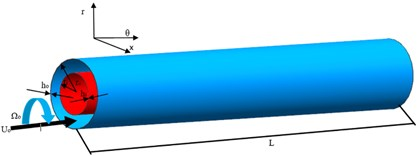### 2.2. Hydrodynamic pressure

Due to the small shell motion, the inviscid fluid forces induced by shell motions are obtained from the potential flow theory. From the impermeability boundary conditions on the shell wall surfaces, the inviscid fluid forces $p$ (the perturbation pressure) on the inner shell or the outer shell are given , because the fluid cannot flow on the interface between the shell and the fluid:

1
${p}_{i}=-\frac{{\rho }_{f}}{\mathrm{\Gamma }}{\left(\frac{\partial }{\partial t}+\frac{{V}_{o}}{r}\frac{\partial }{\partial \theta }+{U}_{o}\frac{\partial }{\partial x}\right)}^{2}\left[{I}_{n}\left(\lambda {r}_{i}\right){K}_{n}^{\text{'}}\left(\lambda {r}_{o}\right)-{I}_{n}^{\text{'}}\left(\lambda {r}_{o}\right){K}_{n}\left(\lambda {r}_{i}\right)\right]{w}_{i},$
2
${p}_{o}=-\frac{{\rho }_{f}}{\mathrm{\Gamma }}{\left(\frac{\partial }{\partial t}+\frac{{V}_{o}}{r}\frac{\partial }{\partial \theta }+{U}_{o}\frac{\partial }{\partial x}\right)}^{2}\left[{I}_{n}^{\text{'}}\left(\lambda {r}_{i}\right){K}_{n}\left(\lambda {r}_{o}\right)-{I}_{n}\left(\lambda {r}_{o}\right){K}_{n}^{\text{'}}\left(\lambda {r}_{i}\right)\right]{w}_{o},$

in which $\Gamma ={I}_{n}^{\text{'}}\left(\lambda {r}_{i}\right){K}_{n}^{\text{'}}\left(\lambda {r}_{o}\right)-{I}_{n}^{\text{'}}\left(\lambda {r}_{o}\right){K}_{n}^{\text{'}}\left(\lambda {r}_{i}\right)$; the mean tangential velocity ${V}_{o}=r×{\mathrm{\Omega }}_{o}$, where ${I}_{n}$ and ${K}_{n}$ are the $n$th-order the first and second modified Bessel functions; the subscripts $i$ and $o$ are the inner shell and the outer shell, respectively; $t$ is the time; the prime indicates the differentiation; $w$ is the $r$-direction displacement; $\lambda$ pertains to the order of axial vibration mode.

### 2.3. The governing equations of motion

Compared with the traditional couple stress theory, the modified couple stress theory proposed by Yang et al.  has been using widely due to its compaction and symmetry. Based on the modified couple stress theory and the Hamilton principle, the shell motions are given:

3
$\frac{\partial {N}_{x}}{\partial x}+\frac{1}{r}\frac{\partial {N}_{\theta x}}{\partial \theta }+\frac{1}{2}\frac{{\partial }^{2}{Y}_{xz}}{r\partial x\partial \theta }+\frac{1}{2}\frac{{\partial }^{2}{Y}_{\theta z}}{{r}^{2}\partial {\theta }^{2}}={\rho }_{s}\frac{{\partial }^{2}u}{\partial {t}^{2}},$
4
$\frac{\partial {N}_{x\theta }}{\partial x}+\frac{1}{r}\frac{\partial {N}_{\theta }}{\partial \theta }-\frac{1}{2}\frac{{\partial }^{2}{Y}_{xz}}{\partial {x}^{2}}-\frac{1}{2}\frac{{\partial }^{2}{Y}_{\theta z}}{r\partial x\partial \theta }={\rho }_{s}\frac{{\partial }^{2}v}{\partial {t}^{2}},$
5
$\frac{{\partial }^{2}{M}_{x}}{\partial {x}^{2}}+\frac{1}{r}\frac{{\partial }^{2}{M}_{x\theta }}{\partial x\partial \theta }+\frac{1}{r}\frac{{\partial }^{2}{M}_{\theta x}}{\partial x\partial \theta }-\frac{{N}_{\theta }}{r}-\frac{1}{r}\frac{{\partial }^{2}{Y}_{x}}{\partial x\partial \theta }+\frac{1}{r}\frac{{\partial }^{2}{Y}_{\theta }}{\partial x\partial \theta }-\frac{1}{2}\frac{{\partial }^{2}{Y}_{x\theta }}{{r}^{2}\partial {\theta }^{2}}+\frac{1}{2}\frac{{\partial }^{2}{Y}_{x\theta }}{\partial {x}^{2}}={\rho }_{s}\frac{{\partial }^{2}w}{\partial {t}^{2}}+{p}_{i/o},$

where ${N}_{ij}$ is the internal forces; ${M}_{ij}$ is the moment resultants; ${\gamma }_{ij}$ is the couple forces; ${T}_{ij}$ is the couple moments; $i$, $j=x$, $\theta$, $r$.

The shell motion equations are given:

6
$Ζ\left[\begin{array}{l}{u}_{i/o}\\ {v}_{i/o}\\ {w}_{i/o}\end{array}\right]=\left[\begin{array}{c}0\\ 0\\ {p}_{i/o}\end{array}\right],$

where $u$, $v$ and $w$ are the $x$-, $\theta$-, and $r$- directions displaces of a shell, respectively; $Ζ$ is the linear matrix operator.

There exists non-zero solutions from the Eq. (6), we arrive at:

7
$\left|Ζ\right|=0.$

We use the zero-level contour method to determine the dispersion relation of $\stackrel{-}{\omega }-{\stackrel{-}{U}}_{o}\left({\stackrel{-}{V}}_{o}\right)\left({\stackrel{-}{V}}_{o}/{\stackrel{-}{U}}_{o}$ is given) by the software package MATLAB. From the feature points of the frequency-fluid velocity curve, we can find the instability velocities and correspond modes.

### 2.4. Validation of the present method

After using the zero-level contour method, the calculated dimensionless results are tabulated in Table 1, and are compared with results of Zhou and Wang .

From the comparison study, the maximum error is 6.0 %. It can be seen that the obtained critical flow velocities in present paper are good agreement with those in published literature.

Table 1Comparisons of critical axial velocities instability by divergence for a simply supported micro shell

 Modified couple stress theory Class theory ${\mathrm{U}}_{\mathrm{o}}$ (m/s) 84.6  85.8 (present) 14.8  13.9 (present) Mode ($m$, $n$) (1, 0) (3, 1) (1, 2) (1, 1)

## 3. Results and discussion

In this paper, the case of elastic outer and perfectly stiff inner shells is considered. We use the simply supported boundary conditions to analyze the dynamical behavior of micro shell. The boundary conditions are given in the form of displaces.

We adopt the following geometry parameters:

The material properties in the calculations are chosen as: $E=$ 1.44×1011 Pa, $\upsilon =$ 0.38, 1000 kgm-3, 1000 kgm-3, $l=h=$ 0.1 μm.

### 3.1. Effects of small size

For the epoxy micro shell in present paper, the definition of the characteristic length is given. $l={b}_{h}/\sqrt{3\left(1-\upsilon \right)}$, where $l$ is the material intrinsic parameter; ${b}_{h}$ is a higher order bending parameter.

The material intrinsic parameter $l$ indeed affects the results. To illustrate the effect of the material intrinsic parameter on the critical velocity, we obtain different $l$ after defining and calculating ${b}_{h}$, as listed in Table 2. Using the same data, the obtained critical velocities by divergence are summarized in Table 3.

Table 2Different values of the material intrinsic parameter l with different bh

 ${b}_{h}$ 0 0.14 0.5 1 $l$ 0 0.1 0.37 0.74 $l\text{'}=l/{r}_{i}$ 0 0.0161 0.0403 0.0807

Table 3Comparisons of dimensionless critical axial velocities by divergence with different l

 $l\mathrm{\text{'}}$ 0 0.0161 0.0403 0.0807 ${\stackrel{-}{U}}_{0}$ 0.00498 0.0060 0.0151 0.0158 modes ($m$, $n$) (1, 1) (1, 2) (1, 2) (1, 2)

It is clear that, the material intrinsic parameter $l$ exerts a very significant influence on the critical axial velocity, which goes up with the increase of $l$. When keeping the length-radius ratio, thickness-radius ratio and gap ratio constant, the relative radius of micro shell ${{r}_{o}}^{\text{'}}={r}_{o}/{r}_{o\mathrm{i}}={r}_{o}/10$ changes from 1 to 50.

Fig. 2 shows the variation of the critical velocities with different relative radii. We found that the material intrinsic parameter effect gradually diminishes when the radius of micro-shell becomes larger enough. To be concluded, the size effect cannot be considered for macro structures.

Fig. 2Variation of dimensionless critical axial flow velocities by divergence with different relative radii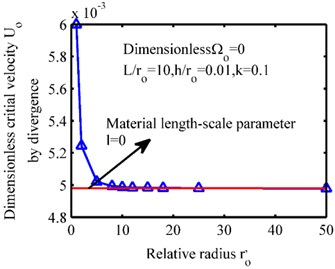Fig. 3Effects of fluid rotation on the instability of the micro shell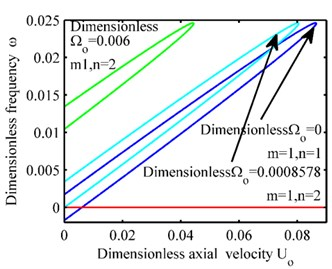### 3.2. Effects of fluid rotation

Fig. 3 depicts the effect of the fluid rotation on the instability behavior of the micro shell. It can be seen that with the increase of the rotating speed of fluid, the critical axial velocity by divergence evidently reduces to zero, which indicating the compound form of divergence and flutter changing into the flutter. We obtain the dimensionless critical angular velocity (0.00086) at the transition point by solving Eq. (7). It should be pointed out that the influence of the angular flow velocity for micro shells is similar to that for macro-shells.

The dimensionless total flow velocity is defined as ${V}_{t}=\sqrt{{\stackrel{-}{U}}_{o}^{2}+{\stackrel{-}{V}}_{o}^{2}}$.

The effect of the fluid rotation on the instability of shell base on the modified coupled stress theory and the classical continuum mechanics theory is illustrated in Fig. 4. From the three curves in the figure, it is seen that the critical total fluid velocities decrease when the ratio of the tangential velocity to the axial velocity increases, and thus the fluid ration lowers the stability of the shell. Furthermore, there appears to be a nonuniform decrease of the total critical flow velocity with the increase of the angular flow velocity. It is also seen that the two curves (for $l=$ 0, or 0.1) present more obvious downward trend while the third curve (for $l=$ 17.6) is declined slowly with increasing of the tangential velocity. To be concluded that, the macro-shell can be affected easily the influences of fluid rotation and the micro shell considering the size effect might be more stable when the material intrinsic parameter $l$ becomes larger.

Fig. 4Effects of the ratio of the tangential velocity to the axial velocity on the critical total flow velocity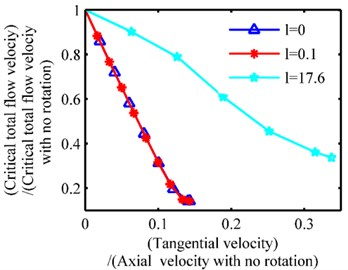Fig. 5Coupling effect of the fluid rotation and size on the system instability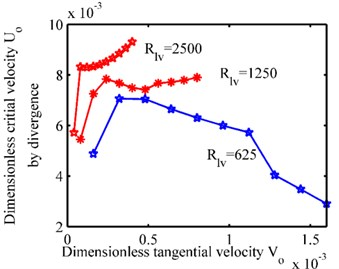### 3.3. Coupling effects of fluid rotation and small size

We define the dimensionless physical parameter ${R}_{lv}=l/{V}_{t}$, which represents the coupling effects of the fluid rotation and small size.

Fig. 5 displays the coupling effects of the fluid rotation and the material intrinsic parameter under different dimensionless parameters ${R}_{lv}$. It is seen that the effects of the fluid rotation or the material intrinsic parameter on the stability of the system are not the same. It is also seen that for a big enough ${R}_{lv}$, the material intrinsic parameter changes have greater influence on the system instability than the fluid rotation. The coupling effects might be considered in industrial applications.

## 4. Conclusions

This paper is concerned with the size-dependent stability analysis of the outer cylindrical shell under the annular flow with two flow velocity components based on the modified couple stress theory by the aid of the Hamilton’s principle. The obtained results indicate that, the fluid rotation lowers the stability of fluid-shell system. Furthermore, when the fluid speed value of rotation increases a certain value, the form of instability could be changed. The obtained results indicate that, the material intrinsic parameter effect results in the fluid-micro shell system more stable. The coupling effect of the small size and fluid rotation on the system stability are evaluated by introducing the new physical coefficient.

#### Acknowledgements

The authors would like to gratefully acknowledge the support provided by the Huai’an natural science research plan project (joint special project) (No. HABL202103). The authors also thank the reviewers for their valuable suggestions.